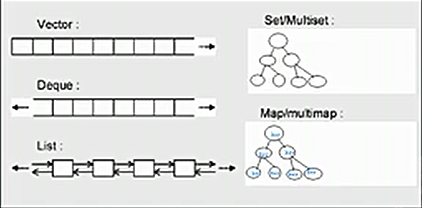STL学习--vector的学习

2015/05/19 19:35

（一）：学习目标：
1：模板，包括函数模板和类模板
2：容器及其分类，以容器中的数据结构
3：容器vector和迭代器的具体用法

（二）：学习
1：函数模板

/** * 学习模板函数 */
int max(int a,int b){
return a > b?a:b;
}

float max(float a,float b){
return a > b?a:b;
}

char max(char a,char b){
return a > b?a : b;
}

/** * 上面函数的模板函数 */
template<typename T>  //或者是 template<class T>
T max(T a,T b){
return a > b?a : b;
}

T代表传入的类型

/* * test.cpp * * Created on: 2015年4月15日 * Author: hongb_000 */
#include <iostream>

using namespace std;

///**
// * 学习模板函数
// */
//int UIP_Max(int a,int b){
// return a > b?a:b;
//}
//
//float UIP_Max(float a,float b){
// return a > b?a:b;
//}
//
//char UIP_Max(char a,char b){
// return a > b?a : b;
//}

/** * 上面函数的模板函数 */

template<typename T>  //或者是 template<class T>
T UIP_Max(T a,T b){
return a > b?a : b;
}

int main(void)
{
//注意这里的<int>是不能省略的，这里表示指定输入的类型
int m = UIP_Max<int>(10,20);
cout << m << endl;

char a = UIP_Max<char>('a','b');
cout << a << endl;

return 0;
}

2：模板类

class IMax{
public:
IMax(int _a,int _b){
a = _a;
b = _b;
}

int GetMax(){
return a>b?a:b;
}

private:
int a;
int b;
};

class FMax{
public:
FMax(float _a,float _b){
a = _a;
b = _b;
}

int GetMax(){
return a>b?a:b;
}

private:
float a;
float b;
};

template <class T> //或者是 template<typename T>
class CMax{
public:
CMax(T _a,T _b){
a = _a;
b = _b;
}

T GetMax(){
return a > b ? a : b;
}

private:
T a;
T b;

};

//这样这个地方的<int>也是不能省略的，为了指定类型
CMax<int> cMax(10,20);
int p = cMax.GetMax();

cout << p << endl;

template<typename T1,typename T2>

3：容器的学习4：vector的学习

1）：vector的简介
vector是将元素置于一个动态的容器中进行管理，相当于一个动态的数组。
vector可以随机访问内部的数据。
vector尾部的插入和移除元素是比较简单的，但是在中间是比较复杂的。

2)：使用前的准备

#include <vector>
using namespace std;

3):默认的构造

vector<T> vec;

vector<int> vec;  //一个存放int类型的vector容器
vector<float> vec; //一个存放float类型的vector

class CA{};
vector<CA*> vecCA;  //用于存放CA的指针
vector<CA> vec;  //用于存放CA的对象，不过这里一定要提供拷贝构造函数

4)：vector末尾的添加和移除操作

vector:push_back(ele); //在容器尾部加入一个元素
vector:pop_back(); //移除容器中的最后一个元素

vector<int> vec;
vec.push_back(1);
//在vector后面插入一个元素
vec.push_back(2);
vec.push_back(3);
vec.push_back(4);
vec.push_back(5);
//将vector的最后一个元素移除

vec.pop_back();

for(size_t i = 0;i < vec.size();i++){
cout << vec.at(i) << " ";
}

1 2 3 4

5)：vector数据的存取

vector获取元素有两种方式：
1：vec.at(i); //但是i越界的话，会跑出out_of_range一场
2：vec[i] //i越界的话没有异常抛出，直接报错

6)：对元素的修改
vector可以直接对某一个位置的元素进行修改。

vce.at(2) = 18;

//位置3的元素现在是3,我们把她改成19

vec.at(2) = 19;
for(size_t i = 0;i < vec.size();i++){ cout << vec.at(i) << " "; }

cout << endl;

1 2 3 4
1 2 19 4

7):访问vector的第一个值和最后一个值
int mFront = vec.front(); //第一个值
int mBack - vec.back(); //最后一个值

vec.front() = 88; //这样就修改成功了

5：迭代器的学习

1）：迭代器的类别

2）：双向迭代器支持的操作

it++,++it,it–,–it,*it,itA=itB,itA==itB,itA!=itB

3）：随机访问存储器

it+=i;it = i;it+i;it[i],itA

vector<int> vecA;
vecA.push_back(1);
vecA.push_back(3);
vecA.push_back(5);
vecA.push_back(7);
vecA.push_back(9);

//注意迭代器的写法
vector<int>::iterator it = vecA.begin();
cout << *it << endl; //输出应该就是第一个元素的值1

++it;  //但是前++要比后++的效率高，因为前++返回的是一个引用，后++返回的是一个值
cout << *it << endl; //输出的应该就是3了

it += 3;
cout << *it << endl; //输出应该就是9了

5）：使用迭代器进行vector的遍历

/** * 使用迭代器进行vector的遍历 */
vector<int>::iterator itA = vecA.begin();

for(;itA != vecA.end();itA++){
cout << *itA << " ";
}

6）：反向迭代器

/** * 反向迭代器,逆序输出 * * 其中的vec.rbegin()指向vector指向最后一个元素 * vec.rbegin()指向vector的第一个元素的上一个位置 */
vector<int>::reverse_iterator rit = vecA.rbegin();

for(;rit != vecA.rend();rit++){
cout << *rit << " ";
}

7）:还有两个

//第一个：vector<T>::const_iterator
//第二个：vector<T>::const_reverse_iterator

6：vector带参数的构造
1）：带迭代器开始和结束的构造

//参数为迭代器的开始和结束

vector<int> vecB(array,array+5);
vector<int> vecC(vecA.begin(),vecA.end());

2）：

vector<int> vecD(3,9);  //vector有3个元素，每个元素都是9

7：vector的赋值
1）：vector:assign(beg,end); //将(beg,end)区间的数据拷贝给本身。左闭右开
2）：vector:assign(n,ele); //n个ele元素
3）：vector:swap(vec); //将vec与本身进行互换

8：vector的大小
1）：vector:size(); //返回容器元素的个数，返回 size_z
2）：vector:empty(); //返回容器是否为空
3）：vector:resize(num); //重新指定容器的长度为num
4）：vector:resize(num,ele); //重新指定容器的长度，超过部分用ele填充，少的部分删除

vector<int> vecE;
vecE.push_back(1);
vecE.push_back(2);
vecE.push_back(3);
//当前vecE有元素1,2,3

vecE.resize(5);  //现在的元素应该为1,2,3,0,0

vecE.resize(8,3); //现在的元素应该为1,2,3,0,0,3,3,3

vecE.resize(2); //现在的元素应该为1,2

8：vector的插入
1）：vector:insert(pos,ele); //在pos位置插入一个ele元素的拷贝，返回新数据的地址
2）：vector:insert(pos,n,ele); //在pos位置插入n个ele元素
3）：vector:insert(pos,beg,end): //在pos插入[beg,end)区间的元素

/** * 插入测试 */
vector<int> vecF;
vecF.push_back(1);
vecF.push_back(3);
vecF.push_back(5);
vecF.push_back(9);

vector<int> vecG;
vecG.push_back(2);
vecG.push_back(4);
vecG.push_back(6);
vecG.push_back(8);

vecF.insert(vecF.begin(),11); //现在vecF中的元素应该是11,1,3,5,7,9
vecF.insert(vecF.begin()+1,2,33); //现在vecF中的元素应该是 11,33,33,1,3,5,9

//执行之后的元素应该是2,4,6,8,11,33,33,1,3,5,9
vecF.insert(vecF.begin(),vecG.begin(),vecG.end());

9：vector的元素删除
1）：vec.clear(); //移除容器中的所有的元素
2）：vec.erase(beg,end): //删除[beg,end)区间的元素
3）：vec.erase(pos); //删除pos位置的元素，返回下一个数据的位置

0
1 收藏

作者的其它热门文章0 评论
1 收藏
0